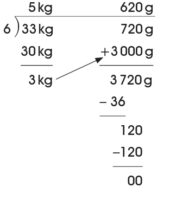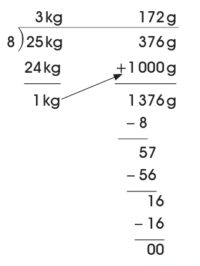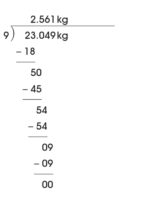# Weight and Its Standard Units

###### TOPIC
• Introduction
• Conversion between Gram and Kilogram
• Addition and Substation of Weight in G and Kg
• Multiplication and Division of Weight in G and Kg
• Word Problems
• Lesson Evaluation

###### INTRODUCTION

Weight is the amount of matter in a body or object.

It measures how light or heavy an object is.

That’s, the different between weight of objects is how an object is lighter or heavier to another.

The standard or metric units of weight are gram (g) and kilogram (kg).

A gram (g) is the unit of weight that’s used to measure lighter objects while a kilogram (kg) is used to measure objects that are heavy.

The relationship between gram and kilogram is,

`1 000 g = 1 kg`

###### CONVERSION OF G TO KG

Working Example 1

Convert 5 000 g to kilograms.

1st, divide the number of gram by 1 000,

5 000 ÷ 1 000 = 5

2nd, change the unit to kilograms,

5 000 g = 5 kg

Working Example 2

Convert 3 765 g to kilograms.

1st, divide the number of gram by 1 000,

3 756 ÷ 1 000 = 3.756

2nd, change the unit to kilograms,

3 756 g = 3.756 kg

Or

3 kg 756 g

Working Example 3

Convert 167 g to kilograms.

1st, divide the number of gram by 1 000,

167 ÷ 1 000 = 0.167

2nd, change the unit to kilograms,

167 g = 0.167 kg

Working Example 4

Convert 13 g to kilograms.

1st, divide the number of gram by 1 000,

13 ÷ 1 000 = 0.013

2nd, change the unit to kilograms,

13 g = 0.013 kg

Working Example 5

Convert 9 g to kilograms.

1st, divide the number of gram by 1 000,

9 ÷ 1 000 = 0.009

2nd, change the unit to kilograms,

9 g = 0.009 kg

WORKING EXERCISES/ASSIGNMENT

Covert the following to kilograms,

1. 0.9 g

2. 135 g

3. 0.048 g

4. 8 787 g

5. 1.25 g

SOLUTIONS

1. 0.9 g = 0.9 x 1 000 = 0.0009 kg

2. 135 g = 135 x 1 000 = 0.135 kg

3. 0.048 g = 0.048 x 1 000 = 0.00048 kg

4. 8 787 g = 8 787 x 1 000 = 8.787 kg

5. 1.25 g = 1.25 x 1 000 = 0.00125

###### CONVERSION OF KG TO G

Working Example 1

Convert 9 kg to grams.

1st, multiply the number of kg by 1 000,

9 x 1 000 = 9 000

2nd, change the unit of grams

9 kg = 9 000 g

Working Example 2

Convert 1.5 kg to grams.

1st, multiply the number of kg by 1 000,

1.5 x 1 000 = 1 500

2nd, change the unit of grams

1.5 kg = 1 500 g

Working Example 3

Convert 0.73 kg to grams.

1st, multiply the number of kg by 1 000,

0.73 x 1 000 = 730

2nd, change the unit of grams

0.73 kg = 730 g

Working Example 4

Convert 5 kg 7 g to grams.

1st, multiply the number of kg by 1 000,

5 x 1 000 + 7 = 5 007

2nd, change the unit of grams

5 kg 7 g = 5 007 g

WORKING EXERCISES/ASSIGNMENT

Complete the following:

1. 7 368 g = ________ kg

2. 9.515 kg = ________ g

3. 1.2 g = ________ kg

4. 1 kg 98 g = ________ kg

5. 1 kg 98 g = ________ g

Working Example 1

Teacher asks each group or pair to add their body weights together.

Lets assume the weight of five pupil in a group are as follows: 25.6 kg, 28.5kg, 27.3 kg, 26.1 kg and 24 kg.

25.6 kg + 28.5kg + 27.3 kg + 26.1 kg + 24 kg =

25.6 kg

+ 28.5kg

+ 27.3 kg

+ 26.1 kg

+ 24.0 kg

______________

131.5 kg

The weight of pupils in group A is 131.5 kg.

Working Example 2

Add 6 kg 950 g to 2 kg 715 g

6 kg 950 g

+ 2 kg 715 g

_____________

9 kg 665 g

Working Example 3

Add 5 kg 56 g and 8 kg 69 g

¹5 kg 56 g

+ 8 kg 59 g

______________

14 kg 05 g

Working Example 4

5.674 kg

+ 3. 475 kg

______________

9.149 kg

Therefore, 9.149 kg = 9.149 x 1 000 = 9 149 g.

WORKING EXERCISES

1. 9 125 g and 6 786 g

2. 4 804 g, 3.498 kg and 2 397 g

3. 989 g and 483 g

4. 2.168 kg + 700 g

5. 6 kg 71 g and 5 kg 92 g

SOLUTIONS

1. 9 125 g and 6 786 g

9 125 g

+ 6 786 g

______________

15 911 g

Therefore, 9 125 g + 6 786 g = 15 911 g or 15 kg 912 g or 15.912 kg

2. 4 804 g, 3.498 kg and 2 397 g

¹4 804 g

(3.498 kg = 3 498 g), + 3 498 g

+ 2 397 g

______________

10 699 g

Therefore, 4 804 g + 3.498 kg + 2 397 g = 10 699 g or 10 kg 699 g or 10.699 kg

3. 989 g and 483 g

¹989 g

+ 483 g

______________

1 472 g

Therefore, 989 g + 483 g = 1 472 g or 1 kg 483 g or 1.472 kg

4. 2.168 kg + 700 g

2.168 kg

(700 g = 0.700 kg) + 0.700 kg

______________

2.868 kg

Therefore, 2.168 kg + 700 g = 2.878 kg or 2 kg 878 g or 2 878 g

5. 6 kg 71 m + 5 kg 92 m

6 kg 71 g

+ 5 kg 92 g

______________

11 kg 163 g

Therefore, 6 kg 71 g + 5 kg 92 g = 11 kg 163 g or 11.163 kg or 11 163 g

###### SUBTRACTION INVOLVING WEIGHT

Working Example 1

Subtract 9 kg 156 g and 8 kg 569 g

9 kg 156 g

– 8 kg 569 g

______________

587 g

Therefore, 9 m 156 cm – 8 m 569 cm = 587 g or 0.587 kg

Working Example 2

4 804 g + 3.498 kg – 2 397 g

4 804 g

(3.498 kg = 3 498 g), + 3 498 g

______________

8 302 g

– 2 397 g

______________

5 905 g

Therefore, 4 804 g + 3.498 kg – 2 397 g = 5 905 g or 5 kg 905 g or 5.905 kg

Working Example 3

989 g – 483 g

989 g

– 483 g

______________

506 g

Therefore, 989 g – 483 g = 506 g or 0.506 kg

Working Example 4

2.168 kg – 700 g

2.168 kg

(700 g = 0.700 kg) – 0.700 kg

______________

1.468 kg

Therefore, 2.168 kg – 700 g = 1.468 kg or 1 kg 468 g

###### MULTIPLICATION OF WEIGHT

Working Example 1

30 kg x 5

3 0

x 5

_______

1 5 0

Therefore, 30 kg x 5 = 150 kg

Working Example 2

170 g x 9

1 7 0

x 9

_______

1 5 3 0

Therefore, 170 g x 9 = 1 530 g

Working Example 3

12.567 kg x 4

12.567

x          4

_______

50.268

Therefore, 12.567 kg x 4 = 50.268 kg.

Working Example 4

31 kg 855 g x 5

31 kg 855 g

x             5

_____________

159 kg 275 g

Therefore, 31 kg 855 g x 5 = 159 kg 275 g.

###### DIVISION OF WEIGHT

Working Example 1

Divide 33 kg 720 g by 6Therefore, 33 kg 720 g ÷ 6 = 5 kg 620 g

Working Example 2

25 kg 376 g ÷ 8Therefore, 25 kg 376 g ÷ 8 = 3 kg 172 g

Working Example 3

23.049 kg ÷ 9Therefore, 23.049 kg ÷ 9 = 2.561 kg

Imagine source – New Method Mathematics Book 4

###### WORD PROBLEMS

Word problems are mathematical problems presented a simple language rather than in mathematical notation.

Working Example 1

The weight of a bag of pure water is 7.15 kg. Some of satchels of water are removed and their weight is 2.68 kg, what is the weight of the remaining satchels?

Solution,

7.15 kg

– 2.68 kg

____________

The weight of the remaining satchels of water is 5.47 kg

Working Example 2

Treasure bought 10 kg 251 g of yams. He bought 8 kg 675 g more. What is the total weight of yams?

Solution,

10 kg 251 g

+ 8 kg 675 g

____________

The total weight of yams is 18 kg 826 g

WORKING EXERCISES

1. Caleb weighs 2.235 kg more than Demilade. If Demilade weighs 84.0 kg, find Caleb’s weight.

2. Caleb weighs 2.235 kg less than Demilade. If Demilade weighs 84.0 kg, find Caleb’s weight.

3. The weight of 5 exercise books is 3.94 kg. 3 of them weigh 2.6 kg. Find the weight of the remaining exercise book.

4. What is 15.38 kg plus 73.4 kg?

5. What is 15.38 kg minus 73.4 kg?

6. What is 15 kg divided by 3?

7. What is 26 g multiply 25?